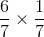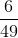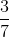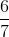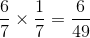HSPT Math : Problem Solving

Example Questions

Example Question #1 : How To Add And Subtract Fractions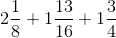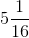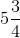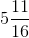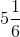Explanation:Convert all mixed numbers to improper fractions.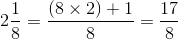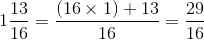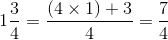Because all of the denominators are not the same find the LCM.  This will become the LCD or Least Common Denominator.

Multiples of 8 are 8,16,24,32.

Multiples of 16 are 16, 32, 48.

Multiples of 4 are 4, 8, 12, 16.

The Least Common Multiple of 8,16 and 4 is 16. The LCD is 16.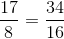The numerator and denominator were both multiplied by 2.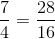The numerator and the denominator were both multiplied by 4.

Now, that all of the fractions have the same denominator, add the numerators.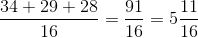Example Question #11 : How To Add And Subtract Fractions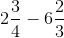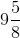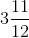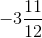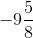Explanation:First, convert all mixed numbers to improper fractions.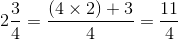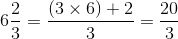Because the denominators are not alike, the fractions have to be converted to equivalent fractions with the same denominator. This is done by finding the LCM which becomes the Least Common Denominator.  The LCD of 4 and 3 is 12.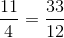Both the numerator and the denominator were multiplied by 3.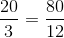Both the numerator and the denominator were multiplied by 4.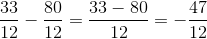The answer is negative because the method to solving would be to subtract and take the sign of the higher number.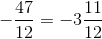Example Question #33 : Problem Solving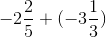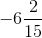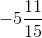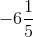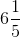Explanation:First, convert all mixed numbers to improper fractions.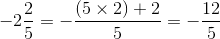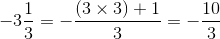Because the denominators are different, find the LCM Of 3 and 5.  This becomes the LCD or least common denominator.

The LCD of 5 and 3 is 15.

Create equivalent fractions with 15 as the denominator.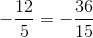Both numerator and denominator were multiplied by 3.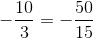Both numerator and denominator were multiplied by 5.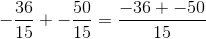When adding two negative integers, the result is negative.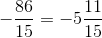Example Question #34 : Problem Solving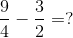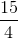Explanation:

To add or subtract fractions, you need a common denominator.

You can multiple the second fraction by two to get a common denominator of four.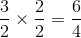Then you just subtract the numerators to get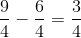.

Example Question #11 : How To Divide Fractions

One Australian dollar is equal to about 86 cents. To the nearest whole, for how many Australian dollars can a tourist to Australia expect to exchange $600 in American money? Possible Answers: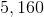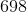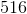Correct answer:Explanation: One Australian dollar is equivalent to 86 cents, or$0.86, in American money, so divide $600 dollars by this conversion factor.$600 is equivalent to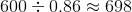Australian dollars.

Example Question #1 : How To Multiply And Divide Fractions

On average, 1 in 9 peanuts that are harvested will only have one pod instead of two pods.  Of those one-pod peanuts, three quarters of them will be smaller than the average peanut.

If 900 peanuts are harvested, how many will be one-pod peanuts that are smaller than average in size?Explanation:

If there are 900 peanuts and 1 in 9 will be one-podded, that means that 100 will be one-podded.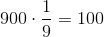Three quarters of these 100 one-podded peanuts will be of smaller than average size. Three quarters of of 100 is 75. Therefore, 75 is the correct answer.

Example Question #36 : Problem Solving

A small bakery sells two flavors of muffins: chocolate and banana.

At the end of the day, there are 13 muffins left.

There arechocolate muffins and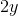banana muffins.

If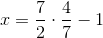how many banana muffins are left?Explanation:

The first step is to solve for x.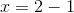If there are 1x chocolate muffins and 2y banana muffins, for a total of 13 muffins then: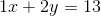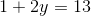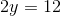Since the number of banana muffins remaining is 2y, there are 12 banana muffins left.

Example Question #1 : How To Multiply And Divide Fractions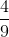of a class of 36 students are boys. If 2 girls and 4 boys were to drop the class, what percentage of the class would be girls?

65%

70%

55%

75%

60%

60%

Explanation:

First determine how many boys and girls are currently in the class.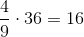boys in the class, which means that there are 20 girls.

When the 6 students drop the new class size will be 30, which will be made up of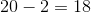girls and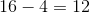boys.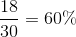Example Question #37 : Problem Solving

How many seconds are in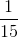of one minute?

5

15

4

30

4

Explanation:

To findof one minute in terms of seconds, you need to first convert one minute to its value in seconds, which is 60 seconds.

Now, you just need to find the value forof 60, which you determine by multiplying the two values: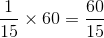You can simplify the above fraction by division, which will you give you your correct answer in seconds: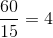Example Question #38 : Problem Solving

Multiply: